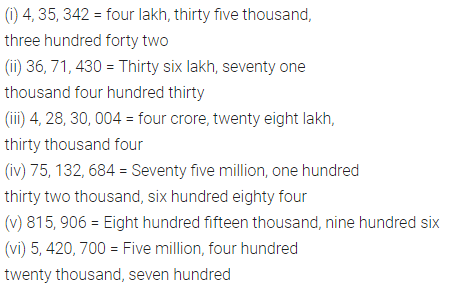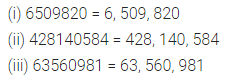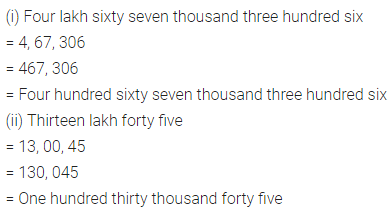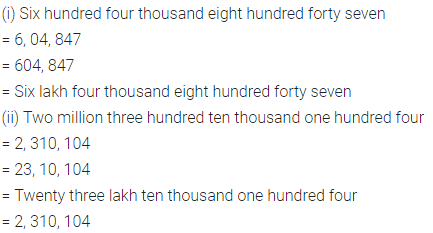# Selina Concise Mathematics Class 6 ICSE Solutions Chapter 3 Numbers in India and International System

## Selina Concise Mathematics Class 6 ICSE Solutions Chapter 3 Numbers in India and International System (With Comparison)

Selina Publishers Concise Mathematics Class 6 ICSE Solutions Chapter 3 Numbers in India and International System (With Comparison)

### Numbers in India and International System Exercise 3 – Selina Concise Mathematics Class 6 ICSE Solutions

Question 1.
Write the following numerals using Indian System words or International system (as required in words) :
(i) 4, 35, 342 = …………..
(ii) 36, 71, 430 = …………..
(iii) 4, 28, 30, 004 = …………..
(iv) 75, 132, 684 = ……………
(v) 815, 906 = …………….
(vi) 5, 420, 700 = ……………..
Solution:Question 2.
Write the following numbers, placing the commas, according to Indian system :
(i) 835629 = …………
(ii) 35640254 = …………
(iii) 2826040 = ………
Solution:Question 3.
Write the following numbers, by placing the commas, according to International system :
(i) 6509820 = ………..
(ii) 428140584 = …………
(iii) 63560981 = ……….
Solution:Question 4.
Fill in the blanks :
(i) Four lakh sixty seven thousand three hundred six.
= ……………… (In numeral form)
= …………… (In International System)
= ……………. (In International numeration)
(ii) Thirteen lakh forty five.
= ……………. (In numeral form)
= ……………. (In International System)
= ……………. (In International numerals)
Solution:Question 5.
Fill in the blanks :
(i) Six hundred four thousand eight hundred forty seven.
= ……………. (In numeral form)
= ……………. (In Indian System)
= ……………… (Number name in Indian System)
(ii) Two million three hundred ten thousand one hundred four
= …………….. (In numeral form)
= …………… (In Indian System)
= ……………. (In Indian numeration)
= …………….. (In international numerals)
Solution: